#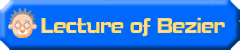## 12.To adjusting a Bezier curve and being complete. ( Procedure 2 of the trace )

It is a sequel in the last time.It adjusts a Bezier curve in Adobe Illustrator.It uses the Direct Selection tool and Pen tool.When adjusting a Bezier curve, too, let's begin at the place to like.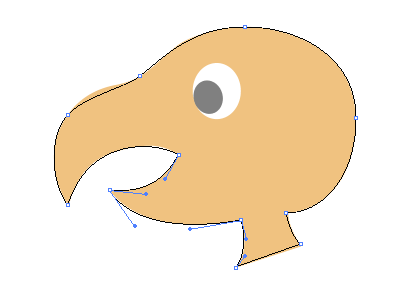( Figure 9 )

The Direct Selection tool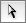from the hit to have begun to draw this timeIt uses and it corrects in turn.

By the segment unit, adjust a control handles on either side and let's take a balance.As for this, the adjustment ended just now to the bill below.( Figure 9 )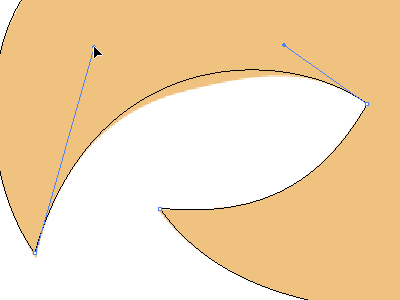( Figure 10 )

It is the inside of the bill above.A center in the segment had been roughly upset when adjusting a control handle with either side, too, and adjusting the neighborhood of the anchor points to the sketch.( Figure 10 )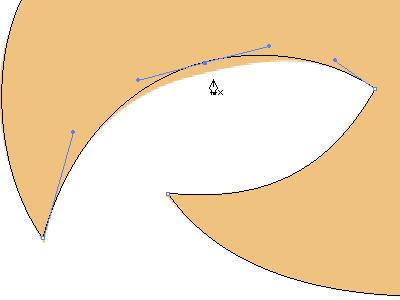( Figure 11 )

Because the pushing off came somewhere however it adjusted control handles, it added an anchor point with the Pen tool.( Figure 11 )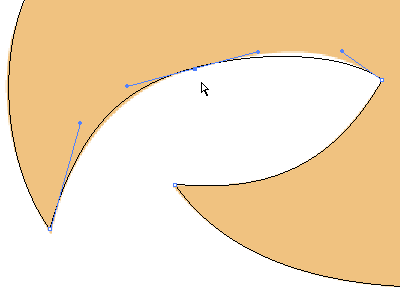( Figure 12 )

Make an anchor point move by the Direct Selection tooland let's adjust a control handle after that.( Figure 12 )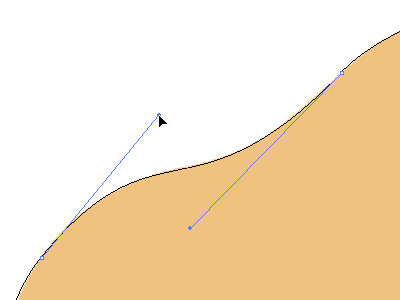( Figure 13 )

This is the base of the bill above ( Figure 13 ).Because the control handles became long, the one which added one anchor point to the center of the segment may be better.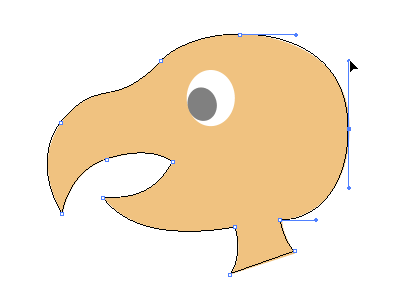( Figure 14 )

It is adjusting the form of the occiput.( Figure 14 )This time, the crack had formed above.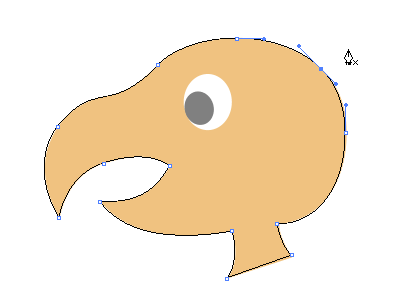( Figure 15 )

It added an anchor point to the part at this crack.( Figure 15 )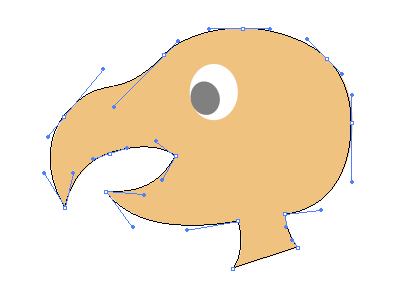( Figure 16 )

It corrected the position of the added anchor points and all adjustments ended.Later, it is completion, drawing eyes.( Figure 16 )

The figure ( figure 17 ) below is in the condition of the end of the 11th lecture, the Bezier curve before adjustment.When attempting to compare, it thinks that it finds how where changed.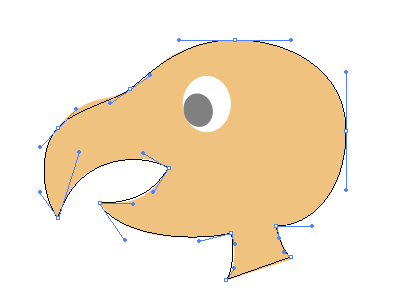( Figure 17 )